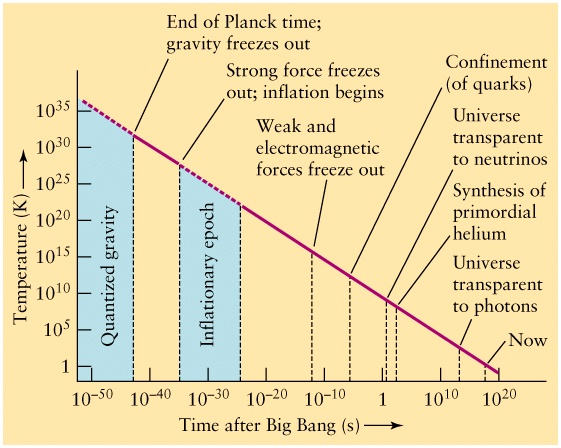# Lecture 32: The Early Universe

## Pair Production and Annihilation

• All types of particles have an anti-matter partner with the exact same mass but opposite charge.
• When matter meets anti-matter, they destroy each other and create two gamma-ray photons with energy

E = m c2

where m is the mass of the particle
• If a photon has energy higher than this threshold, it can create a particle-anti-particle pair.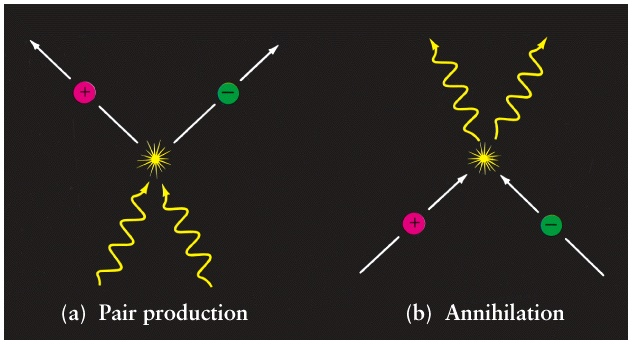• As long as the temperature of the universe is above this energy threshold for the particle of interest, light can create pairs of the particle/anti-particle.
• Below the threshold T, pair production ceases, and the particles annihilate their anti-particle partner.

## Weak Nuclear Reactions

• The weak nuclear reactions transform protons to neutrons and vice versa.
• The two most important weak reactions are:
1. n -> p + e + nu (neutron decay)
2. p + e -> n + nu (electron capture)
• When these reactions can both occur, we have about the same number of neutrons as protons in the universe.
• The electron capture reaction is not favoured because the neutron is more massive than the proton.
• In order to make electron capture occur, we need to supply energy

E = (mn - mp)c2
• Another problem is that we need to have lots of electrons around.
• As long as T is higher than the threshold for creation of electron/positron pairs there will be lots of electrons.
• Both energy thresholds correspond to approximately the same T.
• When the universe cools below this T = 6 x 109 K no more neutrons are produced.
• At this temperature, the abundances of particles are about 6 protons for every 1 neutron.

## Big Bang Nucleosynthesis

• Big Bang Nucleosynthesis is the creation of light elements from the protons and neutrons in the early universe.
• Elements were formed by first creating the simplest possible nucleus and then adding particles to create more complicated nuclei.
• The simplest nucleus is that of Deuterium ( D = 2H) composed of a neutron and a proton.
• n + p -> D + light
• This reaction can't take place if the universe is too hot.
• If a photon has energy larger than
E = ( mn + mp - mD)c2
the photon will break the nucleus into its constituent particles (photodissociation).
• This is called the Deuterium Bottleneck and occurs around T=1010 K.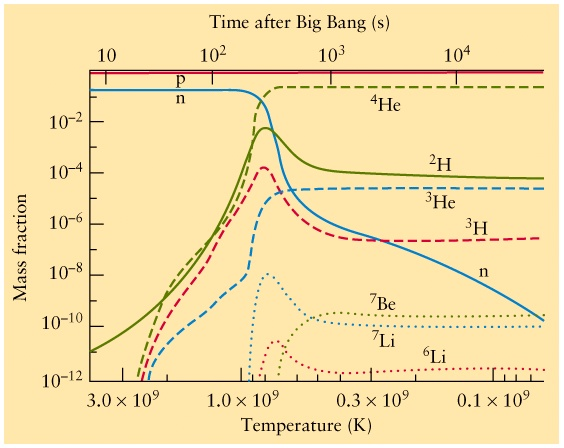• When the universe cools below the Deuterium Bottleneck, Deuterium and heavier elements up to Helium-4 can be synthesized. (Small amounts of Lithium and Beryllium are also produced.)
• The universe is cooling while this happens, so it becomes difficult to produce any element heavier than Be.
• Nucleosynthesis stops at T below 109 K and the abundances of elements are frozen in.
• The figure shows the predicted abundances of light elements.
• These abundances are very similar to the abundances seen in Pop II stars.

## Structure Forms after Big Bang Nucleosynthesis

• After nucleosynthesis finishes the universe is filled with gas that's 75% H and 25% He by mass.
• The density of the gas couldn't have been perfectly smooth.
• Small density fluctuations must have existed.
• Regions that are a little denser than average could collapse if the size of the region is larger than the Jeans length.
• These collapsing regions were probably large enough to create the globular clusters, which then merged to form galaxies.
• When we look at the Cosmic Microwave Background Radiation, we are see the fluctuations in temperature that existed at the time 300,000 years after the Big Bang.
• Regions of lower than average temperature correspond to higher than average density that will collapse later to form globular clusters and galaxies.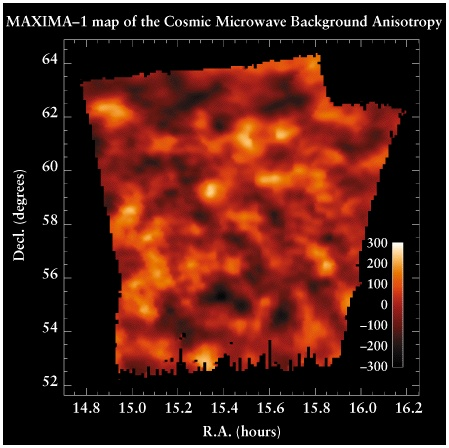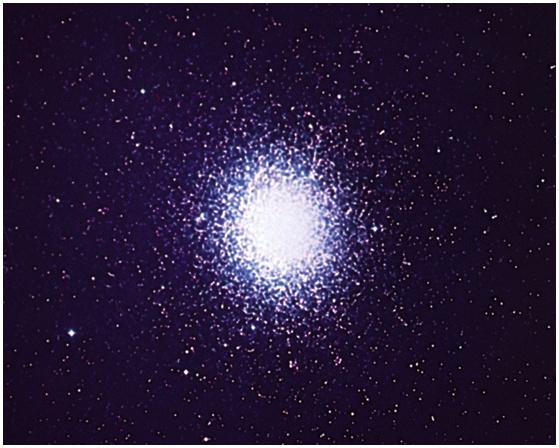## Problems with the Big Bang Theory

1. Why is the Cosmic Microwave Background Radiation so smooth?
• This is called the Horizon Problem.
• Regions in the sky separated by more than about 2 degrees were never in causal contact with each other.
• Why is the temperature of these two regions the same within 1 part in 105?
2. Why is the universe so nearly flat?
• Observations seem to show that the universe has zero curvature.
• But there are many possible values for the curvature, so why this special value?
• This is called the Flatness Problem.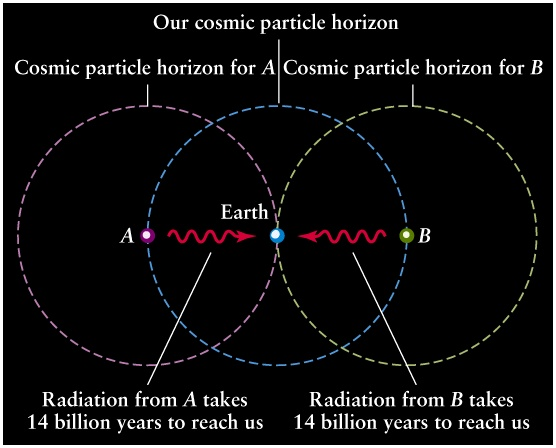## Inflation

• The theory of inflation was proposed to solve these problems.
• Around 10-34 s after t=0, a region of size
r = c t = 3 x 10-26 m
is in causal contact and can be at the same temperature.
• The theory of inflation proposes that at this time a period of exponential expansion occurs lasting until t = 10-32 s.
• During this time the universe increases in size by a factor of e100.
• This causes the region of size 3 x 10-26 m to expand to the size 9 x 1017m.
• This larger region can all be at the same temperature and evolve into the universe which we observe.
• The rapid change in size also has the effect of making the curvature very close to zero.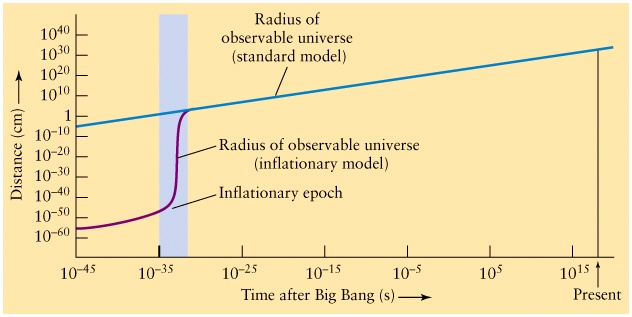## The Initial Singularity

• When the Universe was very young, the distances between things were very tiny.
• Quantum mechanics is needed to describe very tiny distances.
• For instance Heisenberg's uncertainty relation should apply here.
• The equations describing cosmology come out of the theory of general relativity which does not include quantum mechanics.
• The equations of general relativity are only valid when describing distances larger than 10-35m.
• Or equivalently, general relativity only describes the universe when it is older than 10-44 s.
• In order to describe the earliest times, a theory which combines gravity and quantum mechanics is needed (called quantum gravity).
• One theory which might work is called Superstring Theory.
• Superstring theory predicts that the universe is actually 10 dimensional, but that we only directly experience 3 dimensions.
• Superstring theory may be able to describe the earliest times properly.
• This is still unknown territory!